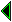## The Bourbaki view

The book series in the name of Nicolas Bourbaki (a pen-name for a group of French mathematicians) 'takes up mathematics from the beginning, and gives complete proofs'. This beginning [bourbaki-sets] is something like Zermelo set theory15 axiomatized in a system of first order logic, which includes an indefinite descriptor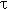building in the Axiom of (Global) Choice.

For whereas in the past it was thought that every branch of mathematics depended on its own particular intuitions which provided its concepts and prime truths, nowadays it is known to be possible, logically speaking, to derive practically the whole of known mathematics from a single source, the Theory of Sets.

Elsewhere [bourbaki-found] says:

On these foundations, I state that I can build up the whole of mathematics of the present day; and if there is anything original in my procedure, it lies solely in the fact that, instead of being content with such a statement, I proceed to prove it in the same way as Diogenes proved the existence of motion; and my proof will become more and more complete as my treatise grows.

The Bourbaki development is intellectually elegant, if rather austere. Stress is placed on developing properties of various key mathematical structures like topological spaces and groups. Some structures are instances of several others (e.g. topological groups are both topological spaces and groups), or are derived from others using certain canonical techniques such as completion. The relative sophistication of these techniques means that the set theory is used heavily even in the study of abstract structures; moreover, set theory is often necessary to provide existence proofs for many of them. So although Hilbert's axiomatic method was an important inspiration, deduction from the structure axioms uses much more than pure (first order) logic. Really, one is working all the time in axiomatic set theory. In the IMPS terminology [farmer-imps] the Bourbaki system is a 'big theory' rather than a mosaic of 'little theories'. A typical example of how concrete structures arise from consideration of abstract ones is the development of real numbers given by [bourbaki-topology1]. The reals are constructed as the completion of the uniform space (this structure is a Bourbaki innovation) induced by the topological group of rationals.

The stated purpose of the Bourbaki treatise is 'to provide a solid foundation for the whole body of modern mathematics'. He works, notionally anyway, with a fixed formal system axiomatizing set theory. [mathias-bourbaki] expresses surprise and dismay that Gödel's theorems on the essential incompleteness of such systems seem not to perturb the Bourbachistes. [kreisel-sad] remarks:

The failure of Hilbert's programme shows that formalism does not provide a theory of the actual process of mathematical reasoning; it is not, so to speak, a fundamental theory. (Of course, formalization is useful, either in limited areas or in combination with other considerations.)

But even Gödel's undecidable sentence for a simple number theory is really just a theoretical pathology. [paris-harrington] have come a bit closer to a realistic mathematical statement that cannot be proved in a simple first order arithmetic; even this relies on a rather artificial encoding of a combinatorial result in number theory. It seems we are nowhere near finding a mathematically interesting incompleteness in ZFC set theory, by which we mean a statement unprovable in ZFC but known for other reasons to be true, as distinct from statements like the Continuum Hypothesis which have no more been decided by non-formalistic methods. Certainly, it's hard to imagine a mainstream mathematical result which could not be proved in ZFC set theory; the Bourbaki project has developed extensive parts of mathematics and failed so far to find any. So from a practical point of view, a disdainful attitude to the significance of Gödel's results seems justified.16 As remarked by [gentzen-investigations]:

It is remarkable that in the whole of existing mathematics only very few easily classifiable and constantly recurring forms of inference are used, so that an extension of these methods may be desirable in theory, but is insignificant in practice.

Although Bourbaki introduces a formal logical system in considerable detail, it is soon forgotten about for reasons of readability and practicality:

If formalized mathematics were as simple as the game of chess, then once our chosen formalized language had been described there would remain only the task of writing out our proofs in this language, [...] But the matter is far from being as simple as that, and no great experience is necessary to perceive that such a project is absolutely unrealizable: the tiniest proof at the beginning of the Theory of Sets would already require several hundreds of signs for its complete formalization. [...] formalized mathematics cannot in practice be written down in full, [...] We shall therefore very quickly abandon formalized mathematics, [...]

Nevertheless, translatability in principle into the formal set theory is taken as the final arbiter of correctness. [bourbaki-sets] clearly says that 'the correctness of a mathematical text is verified by comparing it, more or less explicitly, with the rules of a formalized language'. The intention is that the whole Bourbaki series uses only layers of convention and abbreviation, and could in principle be written out completely formally. This view of formalizability as the criterion of correctness now seems to be widespread in the mathematical community. For example, [maclane-mff] says almost the same thing (p. 377):

As to precision, we have now stated an absolute standard of rigor: A Mathematical proof is rigorous when it is (or could be) written out in the first-order predicate language L(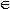) as a sequence of inferences from the axioms ZFC, each inference made according to one of the stated rules. [...] When a proof is in doubt, its repair is usually just a partial approximation to the fully formal version.

That such a view is widespread really does seem to ignore Gödel's theorem. Simple reasoning shows that the Gödel sentence for ZFC set theory is not provable in ZFC, but, if we accept the consistency of ZFC (and surely if it is our criterion for rigour we must), it is true. Are we to dismiss this argument for the truth of the sentence as non-rigorous? In fact [lakatos-proof] identifies this, and other examples like the duality principle of projective geometry, as post-formal proofs, reached by analysis of a formal system rather than derived inside it. (Though of course the reasoning could in its turn be written out in another formal system.) But once again, we accept that Gödel's theorem isn't likely to be relevant to any mathematical proofs we are interested in, and from a strictly practical point of view, this objective standard of rigour is a good one. Rather than discuss that, we want to focus on the following question: why do we only accept formalizability in principle, not formalization in practice?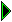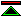John Harrison 96/8/13; HTML by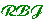96/8/16